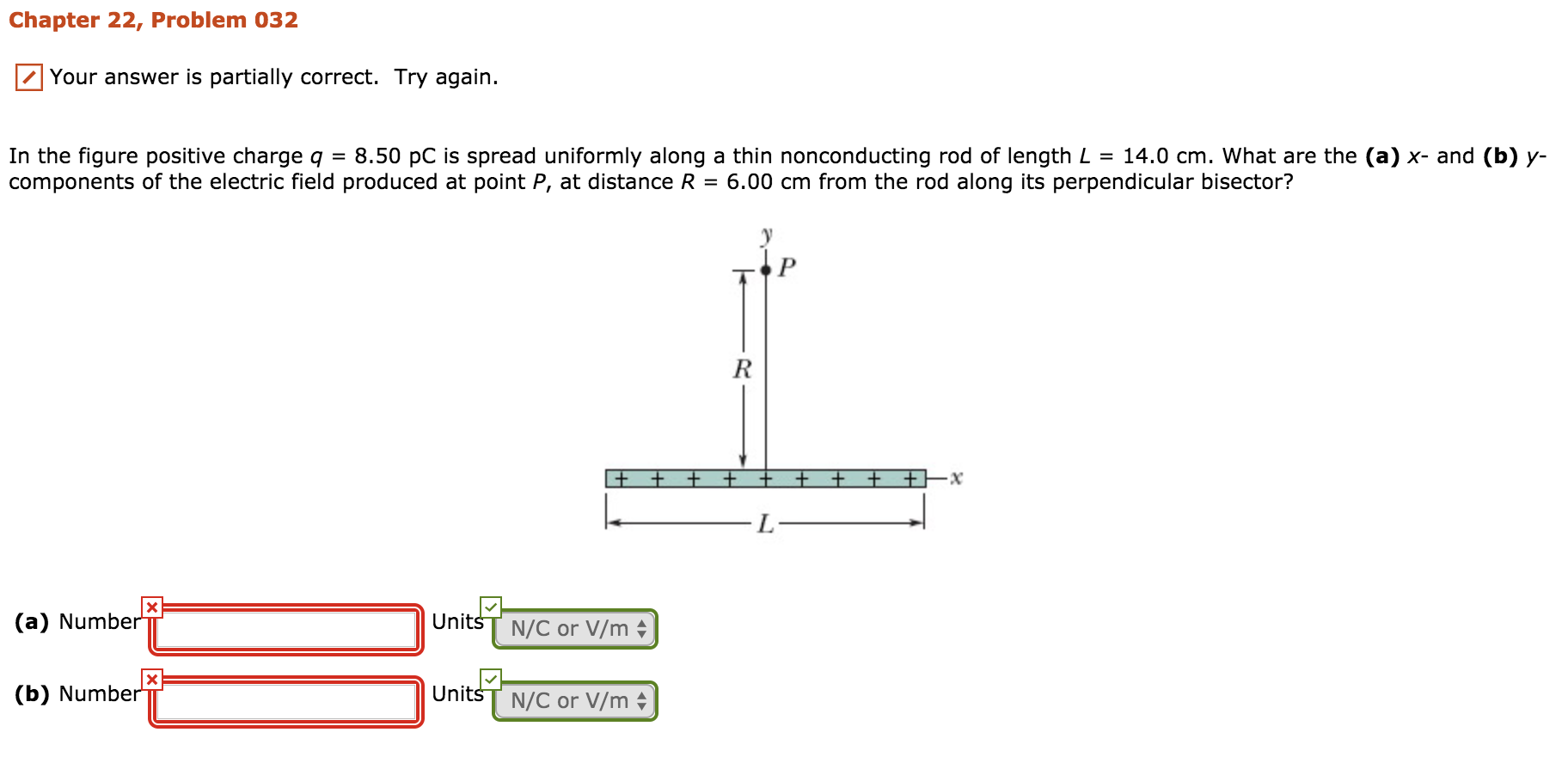# Chapter 22, Problem 032 Your answer is partially correct. Try again. In the figure positive charge q = 8.50 pC is spread uniformly along a thin nonconducting rod of length L 14.0 cm, what are the (a) x-and (b) y- components of the electric field produced at point P, at distance R = 6.00 cm from the rod along its perpendicular bisector? Units (a) Number N/C or V/m UnitsT N/C or V/m

Questionhelp_outlineImage TranscriptioncloseChapter 22, Problem 032 Your answer is partially correct. Try again. In the figure positive charge q = 8.50 pC is spread uniformly along a thin nonconducting rod of length L 14.0 cm, what are the (a) x-and (b) y- components of the electric field produced at point P, at distance R = 6.00 cm from the rod along its perpendicular bisector? Units (a) Number N/C or V/m UnitsT N/C or V/m fullscreen

### Want to see the step-by-step answer?